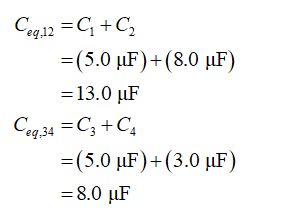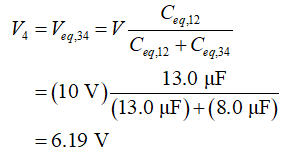Question
1 views

In the figure, V = 10 V, C1 = C4 = 5.0 μF, C2 = 8.0 μF, and C3 = 3.0 μF. What is the charge on capacitor C4?
__________________μC

check_circle

Step 1

In the given circuit, the capacitors C1 and C2 are in parallel combination, and the capacitors C3 and C4 are in parallel combination. Calculate the equivalent capacitance for these two combinations, respectively.Step 2

These two equivalent capacitance are in series now. Calculate the voltage across the equivalent capacitance (Ceq,34), that will be voltage across the capacitor C4....

### Want to see the full answer?

See Solution

#### Want to see this answer and more?

Solutions are written by subject experts who are available 24/7. Questions are typically answered within 1 hour.*

See Solution
*Response times may vary by subject and question.
Tagged in